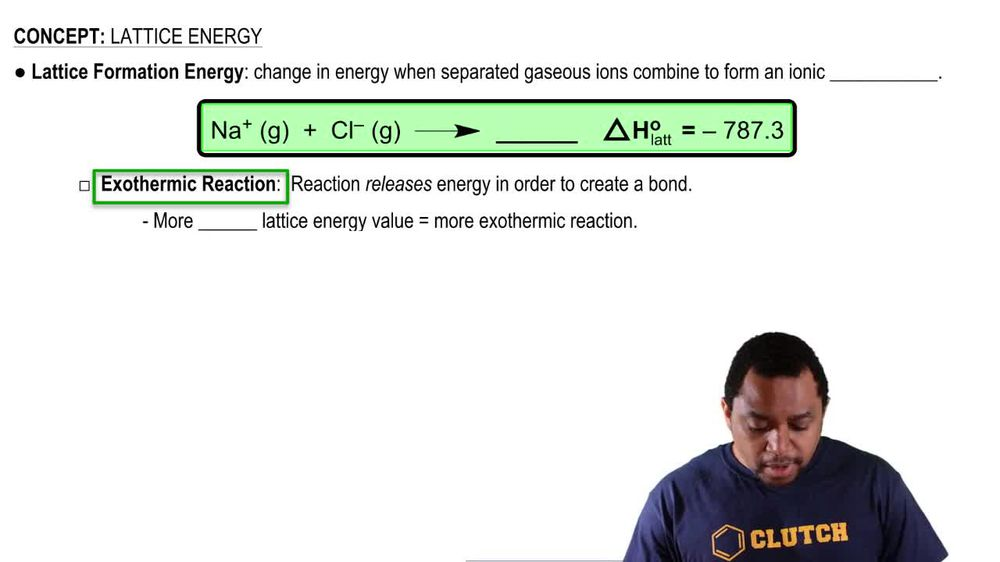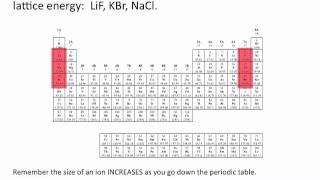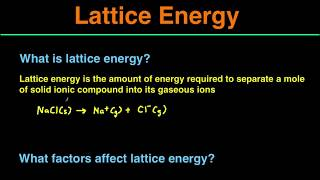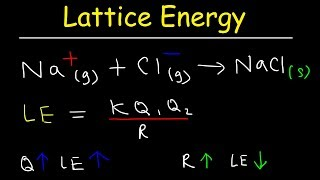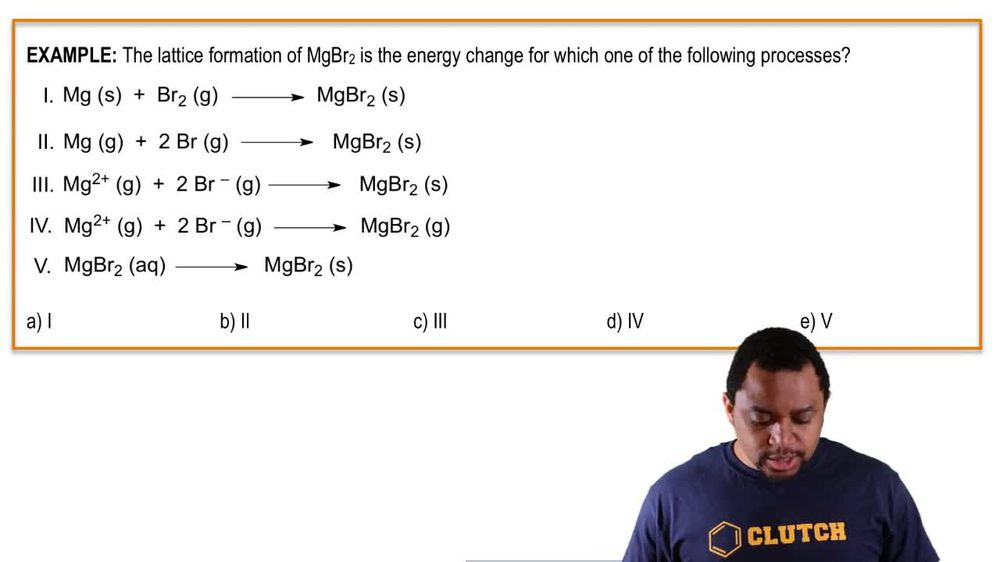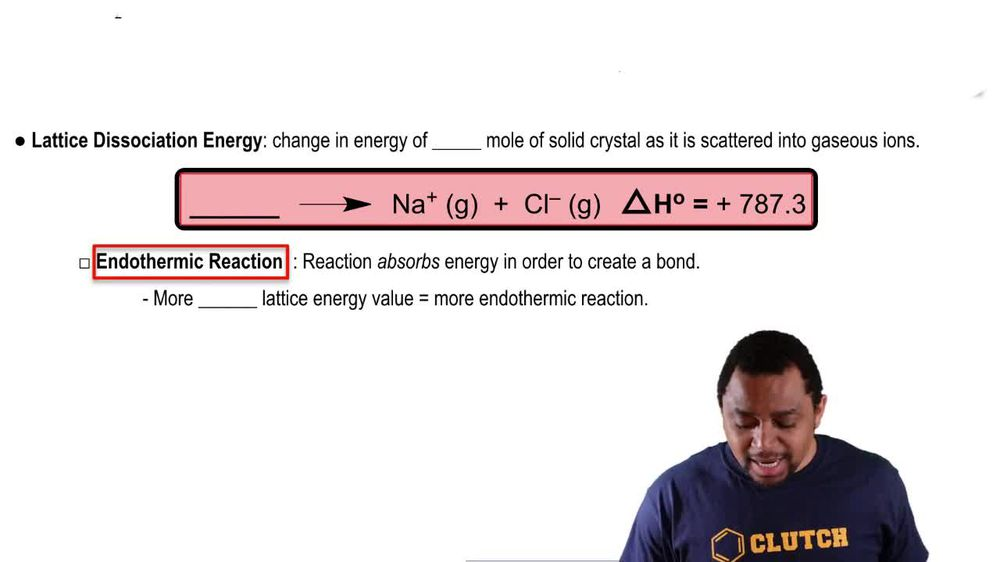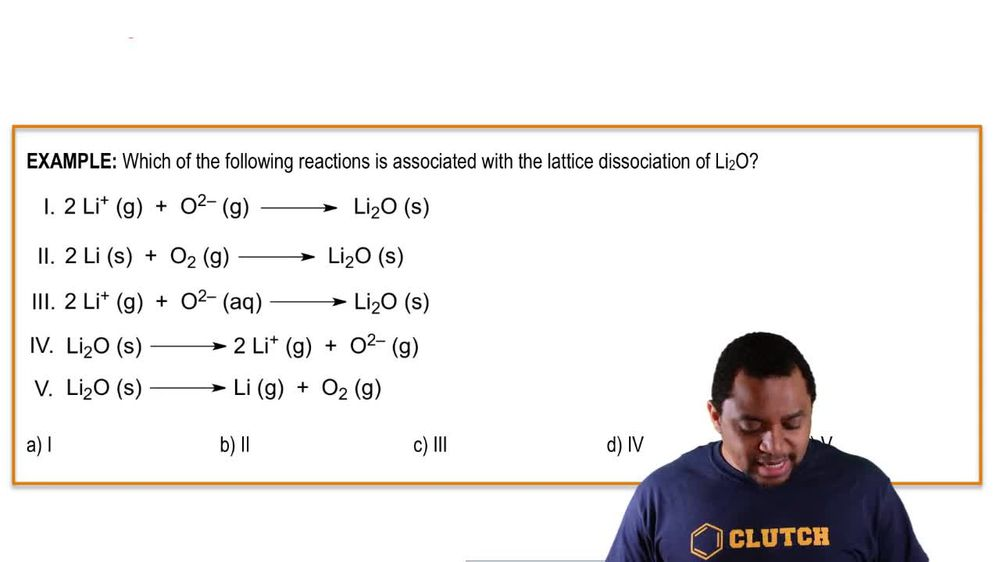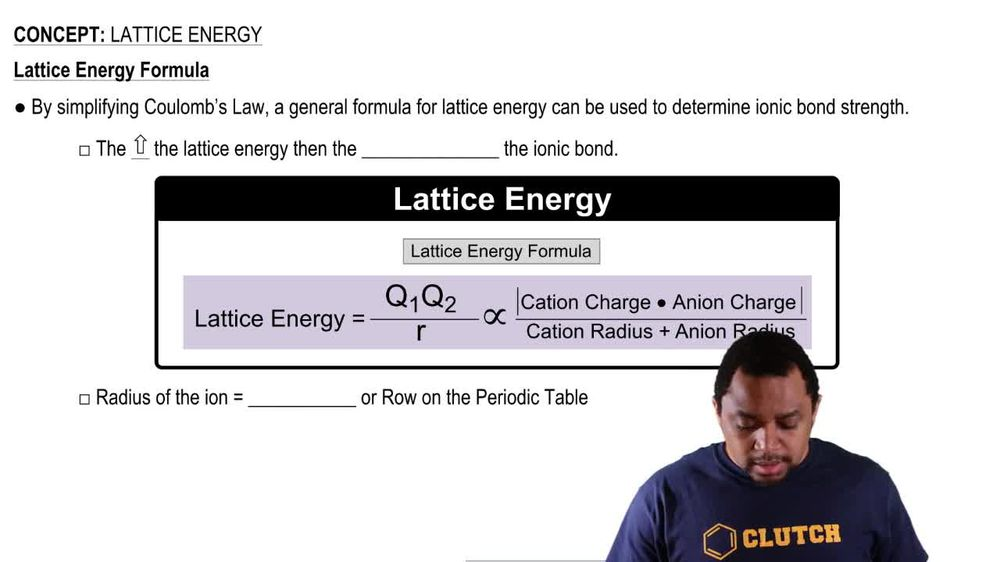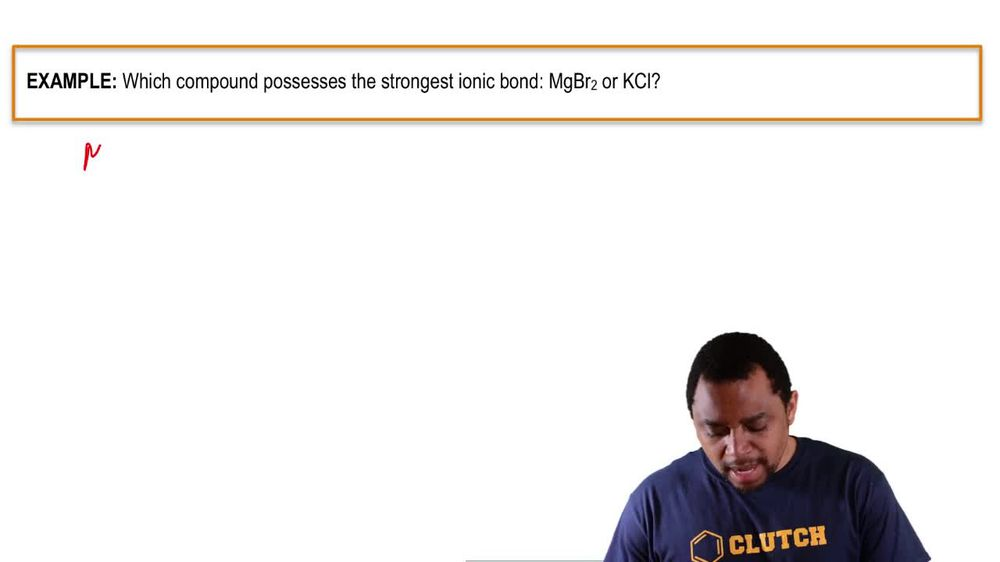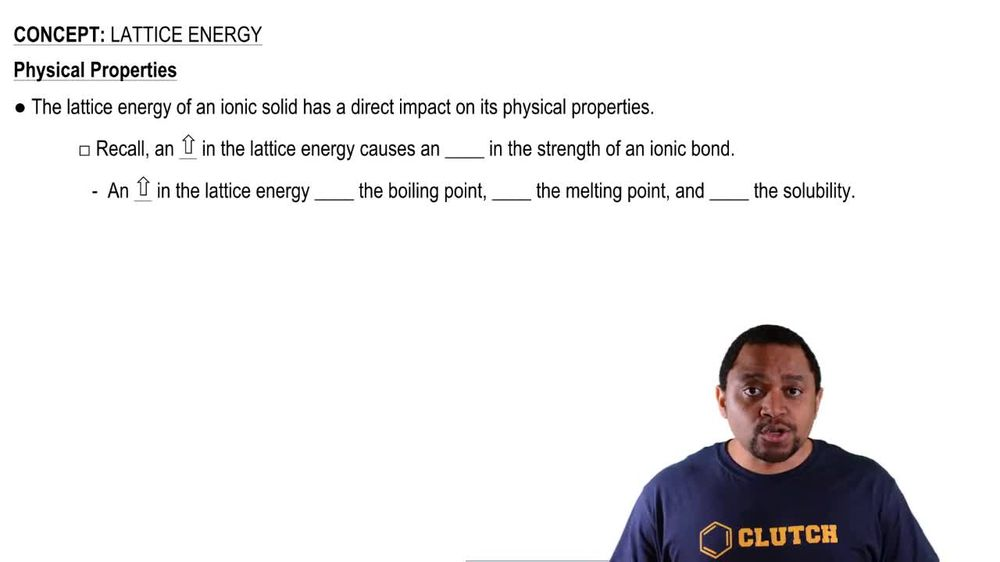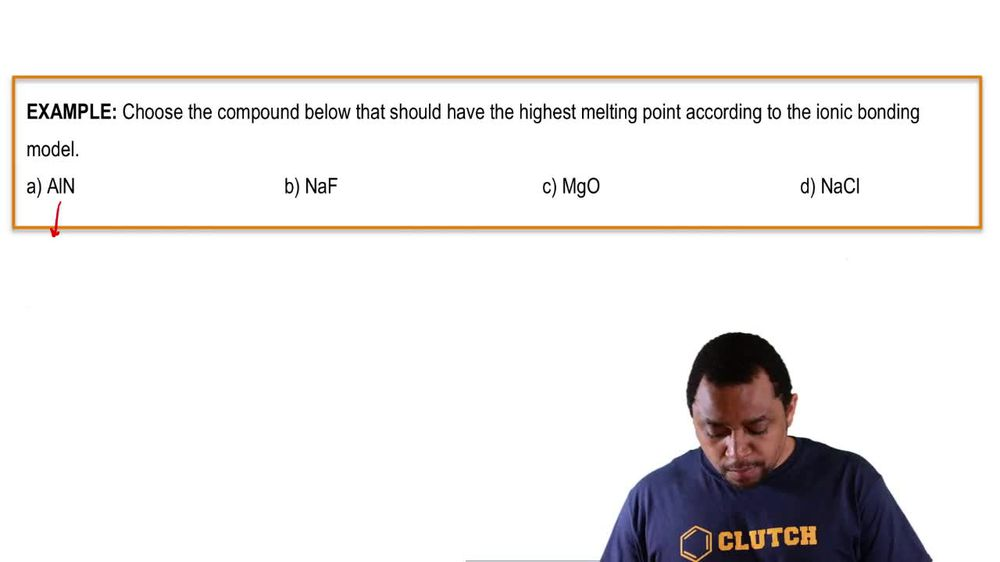Start typing, then use the up and down arrows to select an option from the list.
1. 11. Bonding & Molecular Structure2. Lattice Energy# Lattice Energy

by Jules Bruno
123 views
0
here. We're gonna take a look at the lattice energy formula. We're gonna say by simplifying columns, law. Ah, general formula for lattice energy could be used to determine Ionic bond strength. We're going to say here the higher the lattice energy than the stronger the Ionic bond. Now the formula is lattice. Energy equals Q one overtimes Q two over our This translates into the absolute value. So that means the answer is always gonna be positive. On top of the cat ion charge times the an ion charge and are here is radius. But when we simplify it, it means that we're adding the radius of the cat Ion and the radius of the anti on not realize here that the radius of the ion equals the period or row of the periodic table. So using this simplified lattice energy formula, we can compare different Ionic bonds